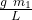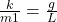## A sphere of mass m1 is attached to an ideal spring, then pulled downward from its equilibrium position and released from rest. A sphere of m

Question

A sphere of mass m1 is attached to an ideal spring, then pulled downward from its equilibrium position and released from rest. A sphere of mass m2 is hung from a massless string of length L, pulled to the right from its equilibrium position, and released from rest. If both spheres have the same period of oscillation, then what is the spring constant of the spring attached to m1

in progress 0
2 months 2021-08-01T07:16:58+00:00 1 Answers 2 views 0

k =Explanation:

In this exercise the two cases presented are a simple harmonic motion, with angular velocity

spring – mass                                      w² = k / m₁

simple pendulum (string- mass m₂)  w² = g / L

angular velocity and period are related

w = 2π/ T

since they indicate that the two periods are equal, the angular velocities are also equal, therefore we can equal the two equationsk =